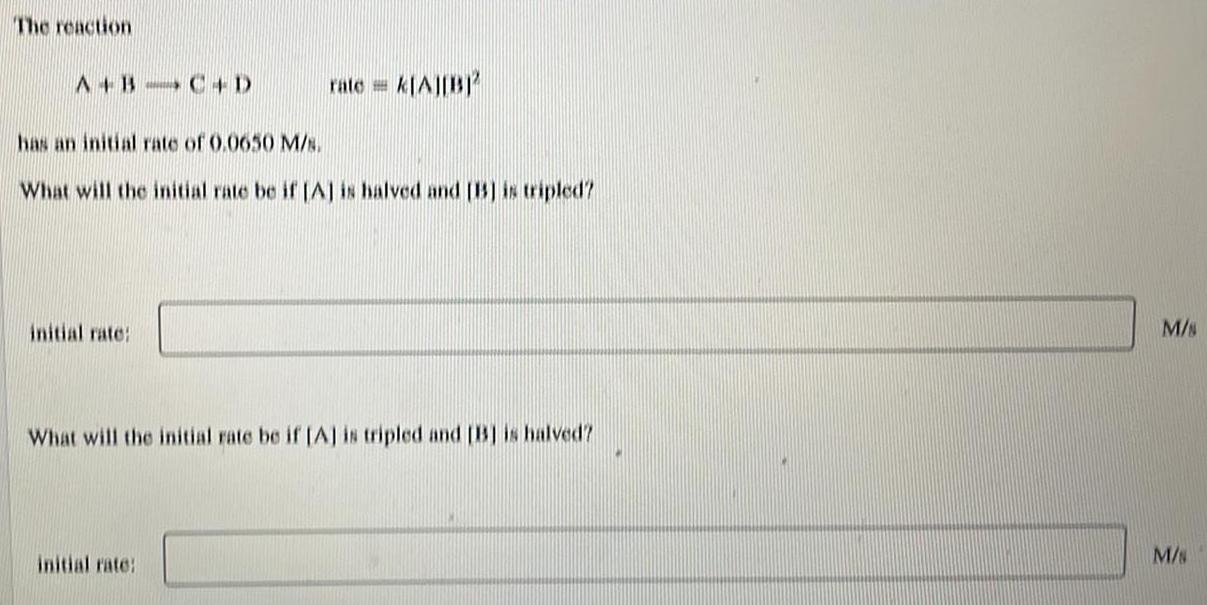Question:

# The reaction A+B --> C+D rate= K[A][B]2 initial rate:

Last updated: 8/9/2022The reaction A+B --> C+D rate= K[A][B]2 initial rate: has an initial rate of 0.0650 M/s. What will the initial rate be if [A] is halved and [B] is tripled? initial rate: What will the initial rate be if [A] is tripled and [B] is halved?